Past Year Questions: Highway Geometric Design & Planning - 2

# Past Year Questions: Highway Geometric Design & Planning - 2 - Notes | Study Transportation Engineering - Civil Engineering (CE)

 1 Crore+ students have signed up on EduRev. Have you?

Question 1. On a circular curve, the rate of superelevation is e. While negotiating the curve a vehicle comes to a stop. It is seen that the stopped vehicle does not slide inwards (in the radial direction). The coefficient of side friction is f. Which of the following is true?    [2015 : 2 Marks, Set-I]
(a) e ≤ f
(b) f < e ≤ 2f
(c) e ≥ 2 f
(d) none of these
(a)
Solution: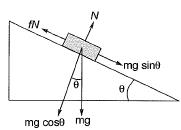For equilibrium,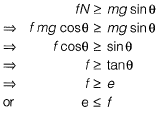Question 2. The acceleration-time relationship for a vehicle subjected to non-uniform acceleration is,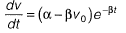where, v is the speed in m/s, t is the time in s, α and β are parameters, and v0 is the initial speed in m/s. If the accelerating behavior of a vehicle, whose driver intends to overtake a slow moving vehicle ahead, is described as,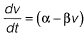Considering α = 2 m/s2, β = 0.05 s-1 and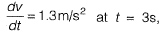the distance (in m) travelled by the vehicle in 35 s is ___________ .     [2015 : 2 Marks, Set-I]
Solution: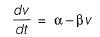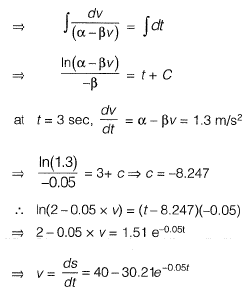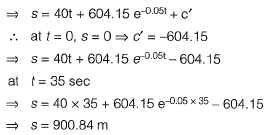Question 3. A sign is required to be put up asking drivers to slow down to 30 km/h before entering Zone Y (see figure). On this road, vehicles require 174 m to slow down to 30 km/h (the distance of 174 m includes the distance travelled during the perception-reaction time of drivers). The sign can be read by 6/6 vision drivers from a distance of 48 m. The sign is placed at a distance of x m from the start of Zone Y so that even a 6/9 vision driver can slow down to 30 km/h before entering the zone. The minimum value of x is________m.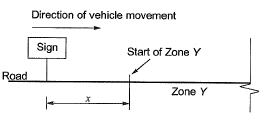[2015 : 2 Marks, Set-I]
Solution:
For a driver having 6/6 vision can see the board from distance 48 m.
For a driver having 6/9 vision can see the board from distance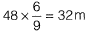Vehicle requires 174 m to slow down to 30 km/hr. So minimum distance of X from the start of Y zone
= 174 - 32 = 142 m.

Question 4. A road is being designed for a speed of 110 km/hr on a horizontal curve with a super elevation of 8%. If the coefficient of side friction is 0.10, the minimum radius of the curve (in m) required for safe vehicular movement is    [2014 : 1 Mark, Set-II]
(a) 115.0
(b) 152.3
(c) 264.3
(d) 528.5
(d)
Solution: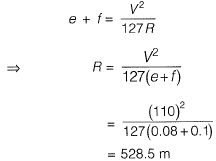Question 5. The perception-reaction time for a vehicle travelling at 90 km/h, given the coefficient of longitudinal friction of 0.35 and the stopping sight distance of 170 m (assume g = 9.81 m/s2), is___________ seconds.    [2014 : 2 Marks, Set-I]
Solution: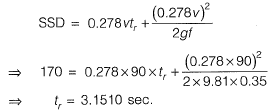Question 6. For a portion of highway descending gradient 1 in 25 meets an ascending gradient 1 in 20. A valley curve needs to be design at a velocity of 90 kmph based on
(i) Head light sight distance equal to stopping sight distance of a level terrain.
Consider length of curve > SSD.
(ii) Comfort condition if rate of change of acceleration is 0.5 m/s3.
Reaction lime = 2.5 sec, coefficient of longitudinal friction [i = 0.35. Height of head light = 0.75 m, and beam angle = 1°.
What is the length of valley curve (in meter) based on comfort condition?    [2013 : 2 Marks]
Solution: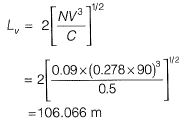Question 7. For a portion of highway descending gradient 1 in 25 meets an ascending gradient 1 in 20. A valley curve needs to be design at a velocity of 90 kmph based on
(i) Head light sight distance equal to stopping sight distance of a level terrain.
Consider length of curve > SSD.
(ii) Comfort condition if rate of change of acceleration is 0.5 m/s3.
Reaction lime = 2.5 sec, coefficient of longitudinal friction [i = 0.35. Height of head light = 0.75 m, and beam angle = 1°.

What is the length of valley curve as per headlight sight distance?   [2013 : 2 Marks]
Solution: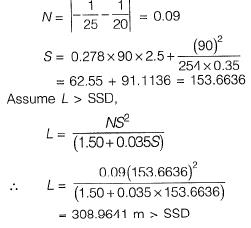Question 8. If v is the initial speed of a vehicle, g is the gravitational acceleration, G is the upward longitudinal slope of the road and fr is the coefficient of rolling friction during braking, the braking distance (measured horizontally) for the vehicle to stop is    [2011 : 2 Marks]
(a)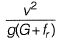(b)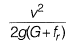(c)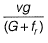(d)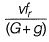Solution: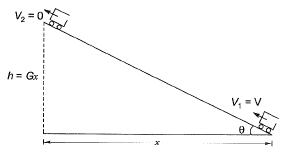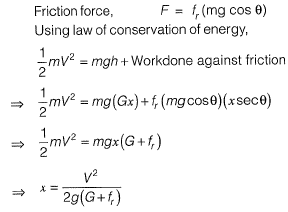Question 9. A vehicle negotiates a transition curve with uniform speed v. If the radius of the horizontal curve and the allowable jerk are R and J, respectively, the minimum length of the transition curve is   [2011 : 1 Mark]
(a) R3/(vJ)
(b) J3/(RV)
(c) v2R/J
(d) v3/(RJ)
(d)

Question 10. The design speed for a two-lane road is 80 kmph. When a design vehicle with a wheelbase of 6.6 m is negotiating a horizontal curve on that road, the off-tracking is measured as 0.096 m. The required widening of carriageway of the two-lane road on the curve is approximately:   [2010 : 2 Marks]
(a) 0.55 m
(b) 0.65 m
(c) 0.75 m
(d) 0.85 m
(c)
Solution: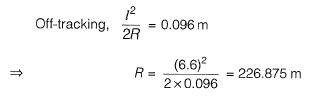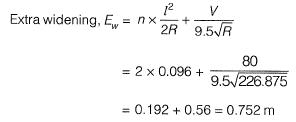Question 11.  Consider the following statements in the context of geometric design of roads:
1. A simple parabolic curve is an acceptable shape for summit curves.
2. Comfort to passengers is an important consideration in the design of summit curves.
The correct option evaluating the above statements and their relationship is     [2010 : 2 Marks]
(a) 1 is true, 2 is false
(b) 1 is true, 2 is true and 2 is the correct reason for 1
(c) 1 is true, 2 is true and 2 is NOT the correct reason for 1
(d) 1 is false and 2 is true
Solution: When fast moving vehicle travels along a summit curve the centrifugal force will act upwards against gravity and hence a part of the pressure on the tyres and spring of the vehicle suspensions is relieved. So there is no problem of discomfort to passengers on summit curves. The only problem is designing summit curves is to provide adequate sight distances.
Circular summit curve is ideal curve as the sight distance available throughout the length of circular curve is constant. The deviation angles in vertical curves of highways are very small and so between the same tangent points, a simple parabola is nearly congruent with a circular arc; also a parabola is very easy for arithmetical manipulation for computing ordinates. Thus, in actual practice a simple parabolic curve is used as a summit curve instead of the circular arc.

The document Past Year Questions: Highway Geometric Design & Planning - 2 - Notes | Study Transportation Engineering - Civil Engineering (CE) is a part of the Civil Engineering (CE) Course Transportation Engineering.
All you need of Civil Engineering (CE) at this link: Civil Engineering (CE)

## Transportation Engineering

22 videos|68 docs|39 tests
 Use Code STAYHOME200 and get INR 200 additional OFF

## Transportation Engineering

22 videos|68 docs|39 tests

Track your progress, build streaks, highlight & save important lessons and more!

,

,

,

,

,

,

,

,

,

,

,

,

,

,

,

,

,

,

,

,

,

;